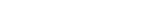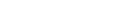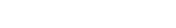xUMACEncyclopedia
In cryptography
Cryptography
Cryptography is the practice and study of techniques for secure communication in the presence of third parties...

, a message authentication code based on universal hashing, or UMAC, is a type of message authentication code
Message authentication code
In cryptography, a message authentication code is a short piece of information used to authenticate a message.A MAC algorithm, sometimes called a keyed hash function, accepts as input a secret key and an arbitrary-length message to be authenticated, and outputs a MAC...

(MAC) calculated choosing a hash function from a class of hash functions according to some secret (random) process and applying it to the message. The resulting digest or fingerprint is then encrypted to hide the identity of the hash function used. As with any MAC, it may be used to simultaneously verify both the data integrity
Data integrity
Data Integrity in its broadest meaning refers to the trustworthiness of system resources over their entire life cycle. In more analytic terms, it is "the representational faithfulness of information to the true state of the object that the information represents, where representational faithfulness...

and the authenticity of a message
Message
A message in its most general meaning is an object of communication. It is a vessel which provides information. Yet, it can also be this information. Therefore, its meaning is dependent upon the context in which it is used; the term may apply to both the information and its form...

. A UMAC has provable cryptographic strength and is usually a lot less computationally intensive than other MACs.

## Universal hashing

Let's say the hash function is chosen from a class of hash functions H, which maps messages into D, the set of possible message digests. This class is called universal
Universal hashing
Using universal hashing refers to selecting a hash function at random from a family of hash functions with a certain mathematical property . This guarantees a low number of collisions in expectation, even if the data is chosen by an adversary...

if, for any distinct pair of messages, there are at most |H|/|D| functions that map them to the same member of D.

This means that if an attacker wants to replace one message with another and, from his point of view the hash function was chosen completely randomly, the probability that the UMAC will not detect his modification is at most 1/|D|.

But this definition is not strong enough — if the possible messages are 0 and 1, D={0,1} and H consists of the identity operation and not, H is universal. But if the digest is then encrypted by modular addition, the attacker can change the message and the digest at the same time and the receiver wouldn't know the difference.

## Strongly universal hashing

A class of hash functions H that is good to use will make it difficult for an attacker to guess the correct digest d of a fake message f after intercepting one message a with digest c. In other wordsneeds to be very small, preferably 1/|D|.
It is easy to construct a class of hash functions when D is field
Finite field
In abstract algebra, a finite field or Galois field is a field that contains a finite number of elements. Finite fields are important in number theory, algebraic geometry, Galois theory, cryptography, and coding theory...

. For example if |D| is prime
Prime number
A prime number is a natural number greater than 1 that has no positive divisors other than 1 and itself. A natural number greater than 1 that is not a prime number is called a composite number. For example 5 is prime, as only 1 and 5 divide it, whereas 6 is composite, since it has the divisors 2...

, all the operations are taken modulo
Modular arithmetic
In mathematics, modular arithmetic is a system of arithmetic for integers, where numbers "wrap around" after they reach a certain value—the modulus....

|D|. The message a is then encoded as an n-dimensional vector over D (a1, a2, ..., an). H then has |D|n+1 members, each corresponding to an (n + 1)-dimensional vector over D (h0, h1, ..., hn). If we letwe can use the rules of probabilities and combinatorics to prove thatIf we properly encrypt all the digests (e.g. with a one-time pad
In cryptography, the one-time pad is a type of encryption, which has been proven to be impossible to crack if used correctly. Each bit or character from the plaintext is encrypted by a modular addition with a bit or character from a secret random key of the same length as the plaintext, resulting...

), an attacker cannot learn anything from them and the same hash function can be used for all communication between the two parties. This may not be true for ECB encryption because it may be quite likely that two messages produce the same hash value. Then some kind of initialization vector
Initialization vector
In cryptography, an initialization vector is a fixed-size input to a cryptographic primitive that is typically required to be random or pseudorandom...

should be used, which is often called the nonce
Cryptographic nonce
In security engineering, nonce is an arbitrary number used only once to sign a cryptographic communication. It is similar in spirit to a nonce word, hence the name. It is often a random or pseudo-random number issued in an authentication protocol to ensure that old communications cannot be reused...

. It has become common practice to set h0 = f(nonce), where f is also secret.

Notice that having massive amounts of computer power does not help the attacker at all. If the recipient limits the amount of forgeries it accepts (by sleeping whenever it detects one), |D| can be 232 or smaller.

## Example

The following C
C (programming language)
C is a general-purpose computer programming language developed between 1969 and 1973 by Dennis Ritchie at the Bell Telephone Laboratories for use with the Unix operating system....

function generates a 24 bit UMAC. It assumes that 'secret' is a multiple of 24 bits, 'msg' is not longer than 'secret' and 'result' already contains the 24 secret bits e.g. f(nonce). nonce does not need to be contained in 'msg'.

#define uchar unsigned char

void UHash24 (uchar *msg, uchar *secret, int len, uchar *result)
{
uchar r1 = 0, r2 = 0, r3 = 0, s1, s2, s3, byteCnt = 0, bitCnt, byte;

while (len-- > 0) {
if (byteCnt-- 0) {
s1 = *secret++;
s2 = *secret++;
s3 = *secret++;
byteCnt = 2;
}
byte = *msg++;
for (bitCnt = 0; bitCnt < 8; bitCnt++) {
if (byte & 1) { /* msg not divisible by x */
r1 ^= s1; /* so add s * 1 */
r2 ^= s2;
r3 ^= s3;
}
byte >>= 1; /* divide message by x */
if (s3 & 0x80) { /* and multiply secret with x, subtracting
the polynomial when necessary to keep its order under 24 */
s3 <<= 1;
if (s2 & 0x80) s3 |= 1;
s2 <<= 1;
if (s1 & 0x80) s2 |= 1;
s1 <<= 1;

s1 ^= 0x1B; /* x^24 + x^4 + x^3 + x + 1 */
}
else {
s3 <<= 1;
if (s2 & 0x80) s3 |= 1;
s2 <<= 1;
if (s1 & 0x80) s2 |= 1;
s1 <<= 1;
}
} /* for each bit in the message */
} /* for each byte in the message */
*result++ ^= r1;
*result++ ^= r2;
*result++ ^= r3;
}